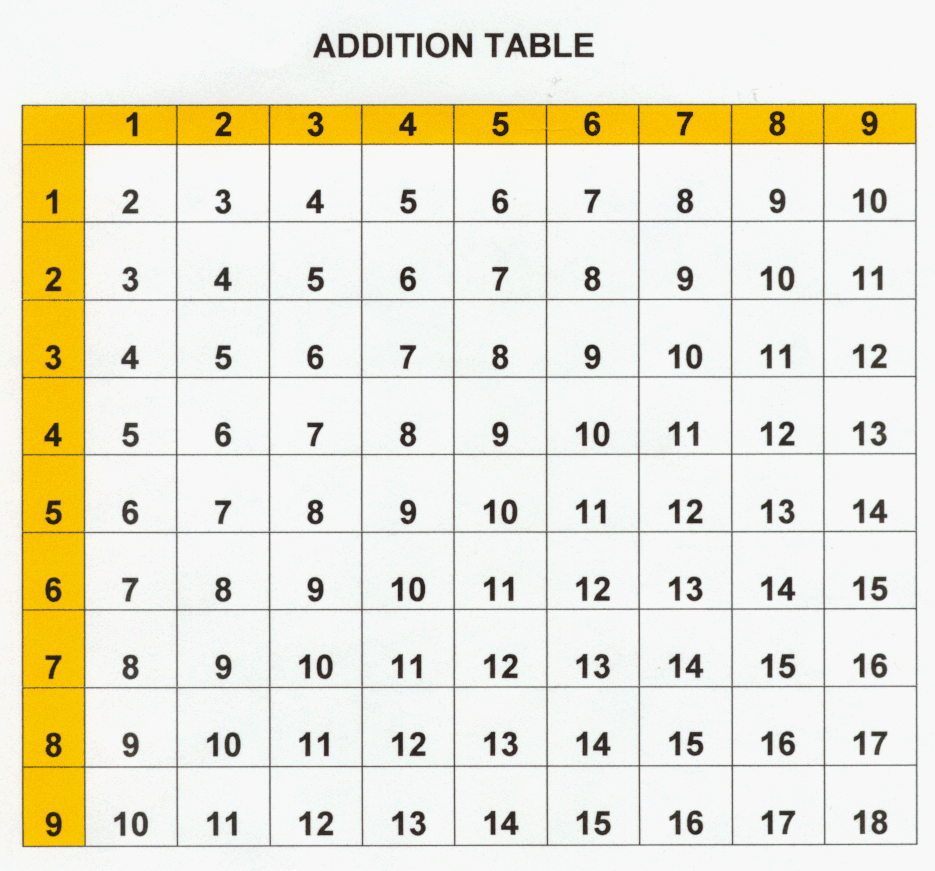top of pageLEARN THE BASICS OF ADDITION AND SUBTRACTION

We aim to help you learn how you sum and subtract numbers together.## NUMBER LINES

To help you understand addition and subtraction, you first need to understand and visualize a number. Each line you see standing out is a number.

Addition and Subtraction: Who We AreNow that you learned how the line chart works, now we learn how we add and subtract.
Lets say you are given 8+4, what you are going to do is locate 8 on the line chart. After that you are going to count one by one starting at 8 and you are going to count one 4 lines after the 8. You should end up with 12.

Addition and Subtraction: Who We Are## Different ways to add and subtract

When adding you can use your fingers to count from a number to another number very easily. For example, if they give you 3 + 5, you can start with 3 fingers. Then count one finger by finger adding one finger until you reach all the five fingers you wanted to add. In the end, you should end with 8 fingers.
The same could be said with subtracting, if they give you 7-4, you first start with 7 fingers then you take away 4 fingers and you should end up with 3 fingers.

## In Detail

When you are going to the right, you are adding numbers. Meaning that numbers get bigger when you go to the right.### Subtraction

When you are going to the left, you are subtracting. Meaning that the numbers get smaller when you go left.

Addition and Subtraction: What We Do## Teaching

When adding, you first have to add the numbers on the right hand side. In this case it would be 3 + 8, which would give you 11. You can't put two digit numbers on the bottom (When you are not yet in the last step). So the next step would be taking the number on the left from the equation we just did, in this case is 1, and you add that number with the remaining numbers on the left, in the problem. In this case it would be 7+1+4, which is 12. You put 12 in the bottom (since it is the last step, you can now put two digit numbers at the bottom in the same time)and you get 121.### 680 + 64

744224

### 870 + 9

879### 153 + 142

295